## Cone-Sphere Intersection

Let a Cone of opening parameterand vertex atintersect a Sphere of Radiuscentered at, with the Cone oriented such that its axis does not pass through the center of the Sphere. Then the equations of the curve of intersection are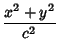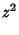(1)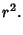(2)

Combining (1) and (2) gives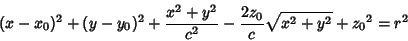(3)(4)
Therefore,andare connected by a complicated Quartic Equation, and,, andby a Quadratic Equation.

If the Cone-Sphere intersection is on-axis so that a Cone of opening parameterand vertex atis oriented with its Axis along a radial of the Sphere of radiuscentered at, then the equations of the curve of intersection are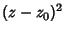(5)(6)

Combining (5) and (6) gives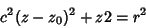(7)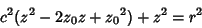(8)(9)(10)(11)